Return
##### Elliptic Spiral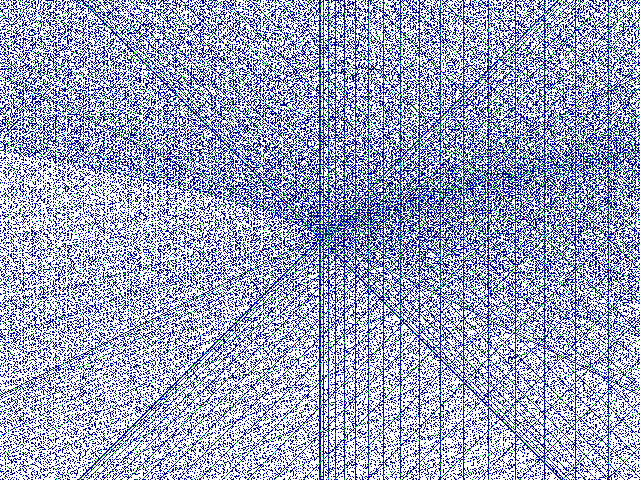Pixels (i,j) are coloured according to number of integer solutions Y,X of Y^2=X^3+i*X+j the center of the image is the origin (i=0,j=0).

If you can extend this image and gain a deeper understanding of how the pixel are calculated then you could be in the line to win a million dollars. See The website of the Clay Mathematics Institute which describes seven millenium problems. This image relates to the Birch Swinnerton Dyer Conjecture.

 This is an example of an elliptic curve.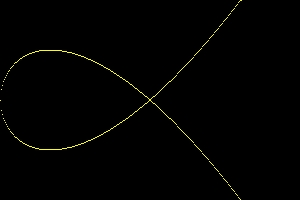A thumbnail of the main image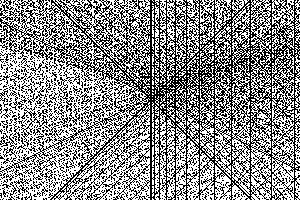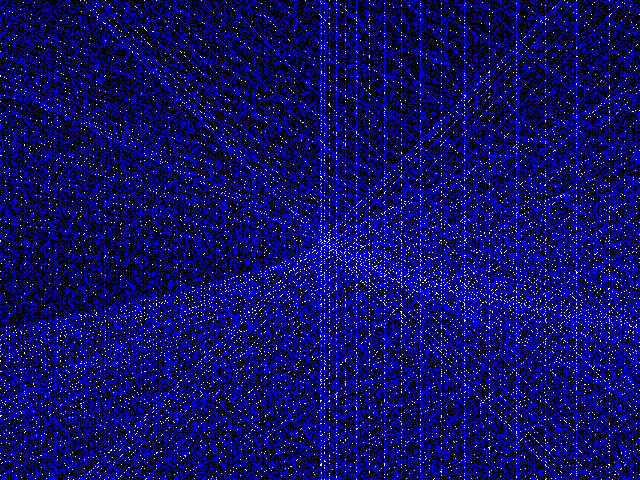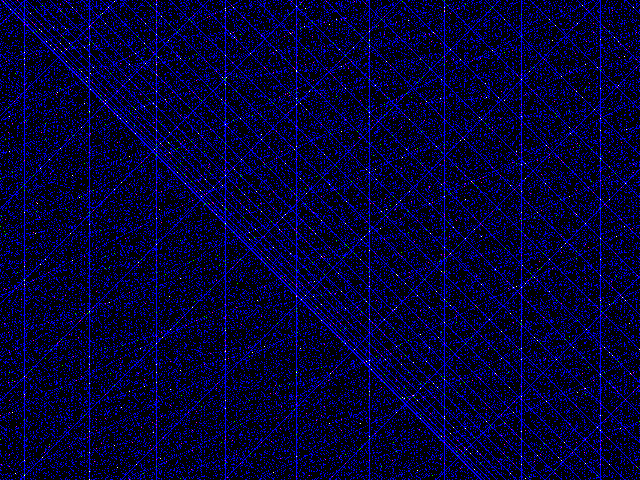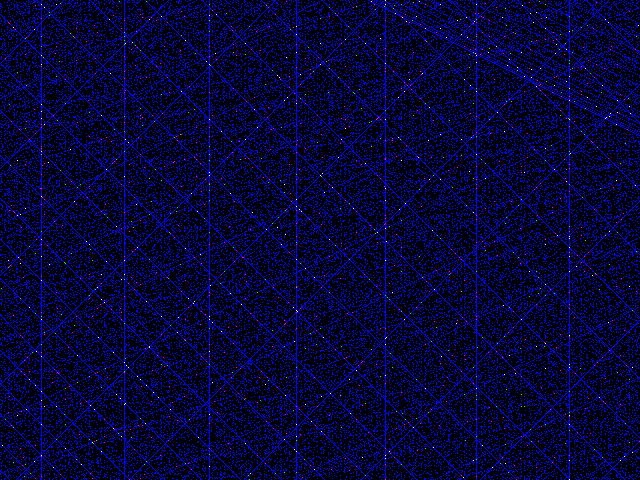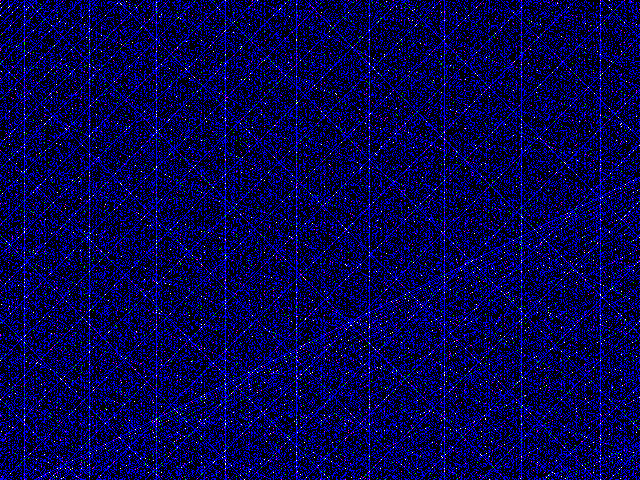Back to the Top